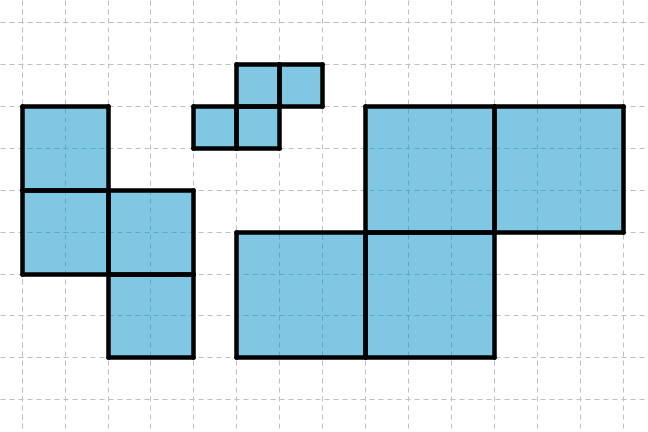## Want to keep learning?

This content is taken from the UNSW Sydney's online course, Maths for Humans: Linear, Quadratic & Inverse Relations. Join the course to learn more.
2.11

## UNSW SydneySame object, different scales, different areas

# Area is a quadratic function

Area is a fundamental measurement in planar geometry, and ends up playing a key role in calculus. It is also a prime example of a quadratic relation under scaling.

In this step, we

• review some basic formulas for areas of shapes

• appreciate the quadratic dependence of area on linear dimension.

## Area scales quadratically with length

If we multiply the sides of a square by two, then the area changes by a factor of four. If we multiply the sides by three, then the area changes by a factor of three squared, or nine. This illustrates that area is a quadratic function of side length, or to put it another way, there is a quadratic relationship between area and side length.In fact it is not necessary to restrict ourselves to squares here: the same law applies to more general rectangles, to triangles, to circles, and indeed to more complicated shapes. If we choose one particular linear measurement to define the size of a two-dimensional shape (such as its diameter), then the area of dilates, or scalings, of that shape will depend quadratically on the values of the linear measurement.

We could say generally that

$\Large{\operatorname{Area}=k \times (\operatorname{Length})^2}.$

Q1 (E): For the Z-shaped shapes in the image at the top of this step, what is the ratio of the area of the largest piece to the smallest piece?

Q2 (M): What is the maximum number of copies of the smallest piece that will fit without overlap into the biggest piece?

## Area formulas for regular figures

For the square the formula is

$\Large \operatorname{Area}=r^2$

where $$\normalsize r$$ is the length of a side. For an equilateral triangle with side length $$\normalsize{r}$$ the formula is

$\Large{\operatorname{Area}=\frac{\sqrt{3}}{4} r^2}.$

In fact for a regular $$\normalsize{n}$$-gon, finding the constant of proportionality relating area and side length is an interesting question, for which approximate answers are all we can expect.

Q3 (M): What is the area of a regular hexagon with side length $$\normalsize{r}$$?

Q4 (C): What is the area of a regular pentagon of radius $$\normalsize{r}$$?For a circle the formula is

$\Large{\operatorname{Area}=\pi r^2}$

where $$\normalsize r$$ is now the radius.

A3. The regular hexagon is composed of six equilateral triangles. If the side length of the hexagon is $$\normalsize{r}$$, then the area will be $$\normalsize{6\times {\frac{\sqrt{3}}{4}r^2}}$$ which is $$\normalsize{3\times {\frac{\sqrt{3}}{2}r^2}}$$. The constant of proportionality is approximately $$\normalsize{2.60}$$.
A4. A regular pentagon is comprised of $$5$$ isosceles triangles, and a bit of trigonometry shows that one of these triangles has area $$\frac{1}{2}r^2 \sin(72^\circ)$$. The area of $$5$$ such triangles is the area of the pentagon. So we have a constant of proportionality of $$\frac{5}{2}\sin(72^\circ)$$, which is approximately $$\normalsize{2.38}$$.
$\Large{\operatorname{Area}= \frac{5}{2}\sin(72^\circ) \times (\operatorname{radius})^2}.$